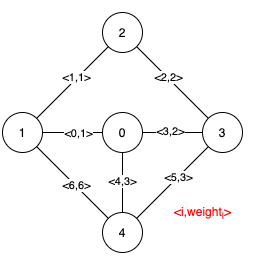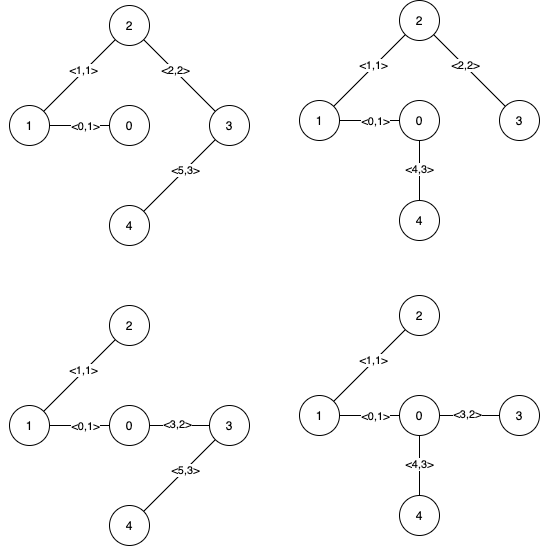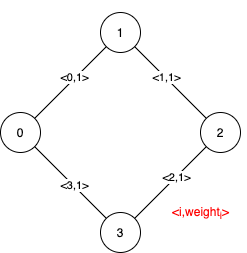Formatted question description: https://leetcode.ca/all/1489.html

# 1489. Find Critical and Pseudo-Critical Edges in Minimum Spanning Tree (Hard)

Given a weighted undirected connected graph with n vertices numbered from 0 to n-1, and an array edges where edges[i] = [fromi, toi, weighti] represents a bidirectional and weighted edge between nodes fromi and toi. A minimum spanning tree (MST) is a subset of the edges of the graph that connects all vertices without cycles and with the minimum possible total edge weight.

Find all the critical and pseudo-critical edges in the minimum spanning tree (MST) of the given graph. An MST edge whose deletion from the graph would cause the MST weight to increase is called a critical edge. A pseudo-critical edge, on the other hand, is that which can appear in some MSTs but not all.

Note that you can return the indices of the edges in any order.

Example 1:Input: n = 5, edges = [[0,1,1],[1,2,1],[2,3,2],[0,3,2],[0,4,3],[3,4,3],[1,4,6]]
Output: [[0,1],[2,3,4,5]]
Explanation: The figure above describes the graph.
The following figure shows all the possible MSTs:Notice that the two edges 0 and 1 appear in all MSTs, therefore they are critical edges, so we return them in the first list of the output.
The edges 2, 3, 4, and 5 are only part of some MSTs, therefore they are considered pseudo-critical edges. We add them to the second list of the output.


Example 2:Input: n = 4, edges = [[0,1,1],[1,2,1],[2,3,1],[0,3,1]]
Output: [[],[0,1,2,3]]
Explanation: We can observe that since all 4 edges have equal weight, choosing any 3 edges from the given 4 will yield an MST. Therefore all 4 edges are pseudo-critical.


Constraints:

• 2 <= n <= 100
• 1 <= edges.length <= min(200, n * (n - 1) / 2)
• edges[i].length == 3
• 0 <= fromi < toi < n
• 1 <= weighti <= 1000
• All pairs (fromi, toi) are distinct.

Related Topics:
Depth-first Search, Union Find

## Solution 1. Kruskal

// OJ: https://leetcode.com/problems/find-critical-and-pseudo-critical-edges-in-minimum-spanning-tree/

// Time: O(ElogE + E^2)
// Space: O(N)
// Ref: https://youtu.be/GzPUvV85kBI
class UnionFind {
vector<int> id;
int size;
public:
UnionFind(int N) : id(N), size(N) {
iota(begin(id), end(id), 0);
}
int find(int x) {
return id[x] == x ? x : (id[x] = find(id[x]));
}
bool connect(int x, int y) {
int p = find(x), q = find(y);
if (p == q) return false;
id[p] = q;
--size;
return true;
}
int getSize() { return size; }
};
class Solution {
int kruskal(int n, vector<vector<int>> &E, int include = -1, int exclude = -1) {
int cost = 0;
UnionFind uf(n);
if (include != -1) {
auto &e = E[include];
uf.connect(e, e);
cost += e;
}
for (int i = 0; i < E.size(); ++i) {
if (i == include || i == exclude) continue;
int u = E[i], v = E[i], w = E[i], id = E[i];
if (!uf.connect(u, v)) continue;
cost += w;
if (uf.getSize() == 1) break;
}
return uf.getSize() == 1 ? cost : INT_MAX;
}
public:
vector<vector<int>> findCriticalAndPseudoCriticalEdges(int n, vector<vector<int>>& E) {
for (int i = 0; i < E.size(); ++i) E[i].push_back(i);
sort(begin(E), end(E), [](vector<int> &a, vector<int> &b) { return a < b; });
int cost = kruskal(n, E);
vector<int> c, p;
for (int i = 0; i < E.size(); ++i) {
if (kruskal(n, E, -1, i) > cost) c.push_back(E[i]);
else if (kruskal(n, E, i) == cost) p.push_back(E[i]);
}
return { c, p };
}
};


Java

class Solution {
public List<List<Integer>> findCriticalAndPseudoCriticalEdges(int n, int[][] edges) {
int edgesCount = edges.length;
List<Integer> indicesList = new ArrayList<Integer>();
for (int i = 0; i < edgesCount; i++)
Collections.sort(indicesList, new Comparator<Integer>() {
public int compare(Integer index1, Integer index2) {
return edges[index1] - edges[index2];
}
});
int minCost = minimumSpanningTreeKruskal(n, indicesList, edges, -1, false);
List<List<Integer>> criticalEdges = new ArrayList<List<Integer>>();
for (int i = 0; i < 2; i++)
for (int i = 0; i < edgesCount; i++) {
if (minimumSpanningTreeKruskal(n, indicesList, edges, i, true) == minCost) {
if (minimumSpanningTreeKruskal(n, indicesList, edges, i, false) > minCost)
else
}
}
return criticalEdges;
}

public int minimumSpanningTreeKruskal(int n, List<Integer> indicesList, int[][] edges, int key, boolean flag) {
int minCost = flag ? edges[key] : 0;
int[] parent = new int[n];
for (int i = 0; i < n; i++)
parent[i] = i;
if (flag)
parent[edges[key]] = edges[key];
int edgesCount = edges.length;
for (int i = 0; i < edgesCount; i++) {
if (indicesList.get(i) != key) {
int parent0 = find(parent, edges[indicesList.get(i)]);
int parent1 = find(parent, edges[indicesList.get(i)]);
if (parent0 != parent1) {
parent[parent0] = parent1;
minCost += edges[indicesList.get(i)];
}
}
}
int first = find(parent, 0);
for (int i = 1; i < n; i++) {
if (find(parent, i) != first)
return Integer.MAX_VALUE;
}
return minCost;
}

public int find(int[] parent, int index) {
while (parent[index] != index) {
parent[index] = parent[parent[index]];
index = parent[index];
}
return index;
}
}